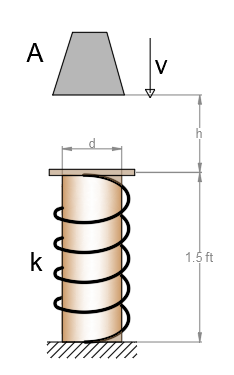# When the 150-lb block is at h=2 ft above the cylindrical post and spring assembly, it has a speed...

## Question:

When the 150-lb block is at h=2 ft above the cylindrical post and spring assembly, it has a speed of v=20 ft/s. If the post is made of 2014-T6 aluminium and the spring has the stiffness of k=220 kip/in, determine the required minimum diameter d of the post to nearest 1/8 in so that it will not yield when it is struck by the block.## Spring force

The spring force is determined by using Hooke's law which tells that spring force and the extension of spring is varies linearly. Mathematically, the spring force is defined as the product of the spring stiffness and the expansion of spring.

## Answer and Explanation: 1

Become a Study.com member to unlock this answer! Create your account

Given data:

• The weight of the block is: {eq}w = 150\;{\rm{lb}} {/eq}.
• The height of the block from the spring is: {eq}h = 2\,{\rm{ft}} {/eq}.
• The...

See full answer below.

#### Learn more about this topic:Hooke's Law & the Spring Constant: Definition & Equation

from

Chapter 4 / Lesson 19
202K

After watching this video, you will be able to explain what Hooke's Law is and use the equation for Hooke's Law to solve problems. A short quiz will follow.